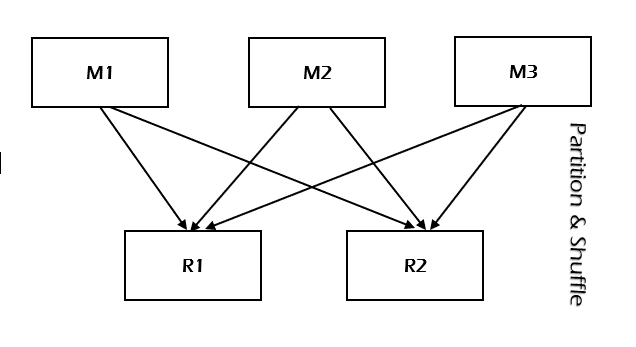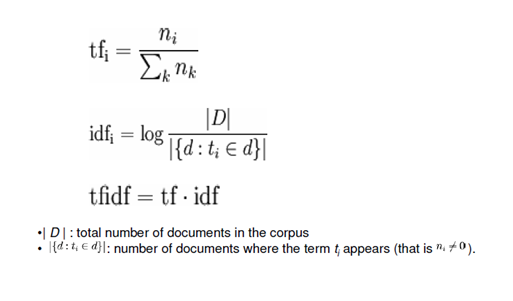###### Summary -

In this topic, we described about the below sections -

• Algorithm Techniques

MapReduce implements many accurate algorithms to divide a task into small parts and assign them to multiple systems. The distinctive MapReduce algorithms including different techniques are -

1. Sorting
2. Searching
3. Indexing
4. Team Frequency – Inverse Document Frequency

## Sorting -

MapReduce is exact suitable for sorting large data sets. Sorting is one of the basic MapReduce algorithms to process and analyze data. MapReduce implements the sorting algorithm to sort the output key-value pairs from Mapper by their keys.

Sorting methods are implemented in the mapper class. The framework groups Mapper outputs based on the keys in this stage. The shuffle and sort phases occur simultaneously after the map-outputs are being fetched.

In the Shuffle and Sort phase, after tokenizing the values in the mapper class, the user-defined (context) class collects the matching valued keys as a collection.

To collect similar intermediate keys, the Mapper class takes the help of RawComparator class to sort the key-value pairs. (key, value) pairs from mappers are sent to a particular reducer based on hash(key).

The set of intermediate key-value pairs is automatically sorted to form key-values (K1, {V11, V21, …}) before they send to the Reducer. In Sorting, Keys are passed to the reducer in sorted order.

#### Algorithm -

Assuming file to be sorted contains lines with single values. Mapper is merely the identity function for the value.

``(k,v) -> (v,_)``

Reducer is the identity function.

``(k,_) -> (k,"")``

Insignificant with single reducer. For multiple reducers, need to choose a partitioning function such as if k1 < k2, partition(k1) <= partition(k2).

(key, value) pairs from mappers are sent to a reducer based on hash(key). Need to pick the hash function for data such as k1 < k2 => hash(k1) < hash(k2).## Searching -

Input is a set of files containing lines of text. The mapper has been passed the pattern to search as a distinctive character. Searching plays a key role in MapReduce algorithm.

It supports in the combiner phase and the Reducer phase. The Map phase processes each input file and provides the data in key-value pairs (<k, v>). The combiner phase (searching technique) will accept the input from the Map phase as a key-value pair.

Using searching technique, the combiner will check to find the key value pair result in each file. The Reducer phase has a chance that required result can find from each file. To avoid redundancy, check all the <k, v> pairs and eliminate duplicate entries if any. The same algorithm is used in between the <k, v> pairs which are from many input files.

#### Algorithm -

1. Mapper compares the line against the pattern.
2. If the pattern matches, mapper outputs (line, _) or (filename+line, _), or …..
3. If the pattern does not match, mapper outputs nothing.
4. Reducer is the identity function.
5. Reducer just outputs each intermediate key.

## Indexing -

The indexing technique is used in MapReduce is known as inverted index. Indexing is used to point a particular data and its address. It performs batch indexing on the input files for a particular Mapper.

#### Assumption -

Input is a set of files containing lines of text. The key is a byte offset of the line, value is the line itself.

#### Algorithm -

The algorithm used in index is inverted index algorithm.

1. Mapper - For each word in the line, emit (word, filename)
Or
For each word in (file, words), map to (word, file)
2. Reducer - Reducer is the Identity function. Collects all values for a given key together.

## Team Frequency – Inverse Document Frequency

TF-IDF is abbreviated as Term Frequency − Inverse Document Frequency. The term 'frequency' refers the number of times a term appears in a document.

TF-IDF is Relevant to text processing and Common web analysis algorithm. TF-IDF is known as term weighting function. TF-IDF assigns a score/weight to each term/word in a document.

TF-IDF very commonly used in text processing and search. TF-IDF main motivation is to measure the relevance of the word. TF-IDF considers both frequency of the word in a given document and the number of documents which contain the word.

#### Term Frequency (TF) -

TF measures frequency of a particular term occurs in a document. While computing TF, all the words are considered equally important. TF is calculated by the number of times a word appears in a document divided by the total number of words in the document.

TF for the Word calculated like below -

``````TF(Word) = (Number of times term the "word"
appears in a document) / (Total number of "word(s)"
in the document)``````

#### Inverse Document Frequency (IDF) -

IDF measures the importance of a word. It is calculated by the number of documents in the text database divided by the number of documents where a specific word appears.

IDF for the Word calculated like below -

``````IDF(word) = log_e(Total number of documents
/ Number of documents with term "word" in it).
``````

#### Algorithm -Information need for the algorithm is -

• Number of times term X appears in a given document.
• Number of terms in each document.
• Number of documents X appears in a given document.
• Total number of documents.